# Sensor: OCM-2

### OCM-2

Select other Sensor.

## Basic information

Name OCM-2 Ocean Colour Monitor scanner 2 8 402-885 0-0 0 0 Indian Oceansat-2 satellite India 0000-00-00 yes

### Bands/Channels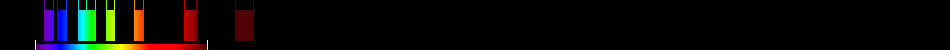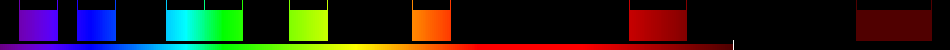Nr. Name Start WL Middle WL End WL Sp.Rg. Spat. Res. Comment
1 402 412 422 20 0
2 433 443 453 20 0
3 480 490 500 20 0
4 500 510 520 20 0
5 545 555 565 20 0
6 610 620 630 20 0
7 725 740 755 30 0
8 845 865 885 40 0

## Indices

Nr. Name Specific Formula Calculated Comment
1 Adjusted transformed soil-adjusted VI $1.22\frac{{8}-1.22{7}-0.03}{1.22{8}+{7}-1.22·0.03+0.08\left(1+{1.22}^{2}\right)}$ Automatic
2 Ashburn Vegetation Index $2.0{8}-{6}$ Automatic
3 Atmospherically Resistant Vegetation Index $\frac{{8}-{7}-{y}\left({7}-{2}\right)}{{8}+{7}-{y}\left({7}-{2}\right)}$ Automatic
4 Atmospherically Resistant Vegetation Index 2 $-0.18+1.17\left(\frac{{8}-{7}}{{8}+{7}}\right)$ Automatic
5 Average reflectance 750 to 850 ${\mathrm{Averagereflectancebetween7and8}}$ Automatic
6 Blue-wide dynamic range vegetation index $\frac{0.1{8}-{2}}{0.1{8}+{2}}$ Automatic
7 Canopy Chlorophyll Content Index $\frac{\frac{{8}-{7}}{{8}+{7}}}{\frac{{8}-{\mathrm{Red}}}{{8}+{\mathrm{Red}}}}$ Automatic
8 Chlorophyll Index Green $\frac{{8}}{{4}}-1$ Automatic
9 Chlorophyll IndexRedEdge $\frac{{8}}{{7}}-1$ Automatic
10 Chlorophyll vegetation index ${8}\frac{{7}}{{{4}}^{2}}$ Automatic
11 Coloration Index $\frac{{7}-{2}}{{7}}$ Automatic
12 Corrected Transformed Vegetation Index $\frac{\left(\frac{{7}-{4}}{{7}+{4}}\right)+0,5}{|\left(\frac{{7}-{4}}{{7}+{4}}\right)+0,5|}·\sqrt{|\left(\left(\frac{{7}-{4}}{{7}+{4}}\right)\right)+0,5|}$ Automatic
13 CRI550 ${{4}}^{\left(-1\right)}-{{5}}^{\left(-1\right)}$ Automatic
14 Difference NIR/Green ${8}-{4}$ Automatic
15 Differenced Vegetation Index MSS $2.4{8}-{6}$ Automatic
16 Enhanced Vegetation Index $2.5\frac{{8}-{7}}{\left({8}+6{7}-7.5{2}\right)+1}$ Automatic
17 Enhanced Vegetation Index 2 $2.4\frac{{8}-{7}}{{8}+{7}+1}$ Automatic
18 Enhanced Vegetation Index 2 $2.5\frac{{8}-{7}}{{8}+2.4{7}+1}$ Automatic
19 Ferric iron, Fe3+ $\frac{{6}}{{5}}$ Automatic
20 Global Environment Monitoring Index $\left(\frac{2\left({{8}}^{2}-{{7}}^{2}\right)+1.5{8}+0.5{7}}{{8}+{7}+0.5}\left(1-0.25\frac{2\left({{8}}^{2}-{{7}}^{2}\right)+1.5{8}+0.5{7}}{{8}+{7}+0.5}\right)-\frac{{7}-0.125}{1-{7}}\right)$ Automatic
21 Green atmospherically resistant vegetation index $\frac{{8}-\left({4}-\left({2}-{7}\right)\right)}{{8}-\left({4}+\left({2}-{7}\right)\right)}$ Automatic
22 Green leaf index $\frac{2{4}-{7}-{2}}{2{4}+{7}+{2}}$ Automatic
23 Green Normalized Difference Vegetation Index $\frac{{8}-{5}}{{8}+{5}}$ Automatic
24 Green Optimized Soil Adjusted Vegetation Index $\frac{{8}-{4}}{{8}+{4}+{Y}}$ Automatic
25 Green Soil Adjusted Vegetation Index $\frac{{8}-{4}}{{8}+{4}+{L}}\left(1+{L}\right)$ Automatic
26 Green-Blue NDVI $\frac{{8}-\left({4}+{2}\right)}{{8}+\left({4}+{2}\right)}$ Automatic
27 Green-Red NDVI $\frac{{8}-\left({4}+{7}\right)}{{8}+\left({4}+{7}\right)}$ Automatic
28 Hue $\mathrm{arctan}\left(\frac{2{7}-{4}-{2}}{30.5}\left({4}-{2}\right)\right)$ Automatic
29 Ideal vegetation index $\frac{{8}-{b}}{{a}·{7}}$ Automatic
30 Infrared percentage vegetation index $\frac{\frac{{8}}{{8}+{7}}}{2}\left(\left(\frac{{7}-{4}}{{7}+{4}}\right)+1\right)$ Automatic
31 Intensity $\left(\frac{1}{30.5}\right)\left({7}+{4}+{2}\right)$ Automatic
32 Inverse reflectance 550 ${{5}}^{\left(-1\right)}$ Automatic
33 Log Ratio $\mathrm{log}\left(\frac{{8}}{{7}}\right)$ Automatic
34 mCRIG $\left({{4}}^{\left(-1\right)}-{{5}}^{\left(-1\right)}\right)·{8}$ Automatic
35 Misra Green Vegetation Index $-0.386{5}-0.530{6}+0.535{7}+0.532{8}$ Automatic
36 Misra Non Such Index $0.404{5}-0.039{6}-0.505{7}+0.762{8}$ Automatic
37 Misra Soil Brightness Index $0.406{5}+0.600{6}+0.645{7}+0.243{8}$ Automatic
38 Misra Yellow Vegetation Index $0.723{5}-0.597{6}+0.206{7}-0.278{8}$ Automatic
39 Modified Simple Ratio NIR/RED $\frac{\left(\frac{{8}}{{7}}\right)-1}{\sqrt{\left(\frac{{8}}{{7}}\right)+1}}$ Automatic
40 Modified Soil Adjusted Vegetation Index $\frac{2{8}+1-\sqrt{{\left(2{8}+1\right)}^{2}-8\left({8}-{7}\right)}}{2}$ Automatic
41 Nonlinear vegetation index $\frac{{{8}}^{2}-{7}}{{{8}}^{2}+{7}}$ Automatic
42 Norm G $\frac{{4}}{{8}+{7}+{4}}$ Automatic
43 Norm NIR $\frac{{8}}{{8}+{7}+{4}}$ Automatic
44 Norm R $\frac{{7}}{{8}+{7}+{4}}$ Automatic
45 Normalized Difference 415/435 $\frac{{1}-{2}}{{1}+{2}}$ Automatic
46 Normalized Difference 550/450 $\frac{{5}-{2}}{{5}+{2}}$ Automatic
47 Normalized Difference 750/550 $\frac{{7}-{5}}{{7}+{5}}$ Automatic
48 Normalized Difference Green/Red $\frac{{4}-{7}}{{4}+{7}}$ Automatic
49 Normalized Difference NIR/Blue $\frac{{8}-{2}}{{8}+{2}}$ Automatic
50 Normalized Difference NIR/Green $\frac{{8}-{4}}{{8}+{4}}$ Automatic
51 Normalized Difference NIR/Rededge $\frac{{8}-{7}}{{8}+{7}}$ Automatic
52 Normalized Difference Red/Green $\frac{{7}-{4}}{{7}+{4}}$ Automatic
53 Pan NDVI $\frac{{8}-\left({4}+{7}+{2}\right)}{{8}+\left({4}+{7}+{2}\right)}$ Automatic
54 Red-Blue NDVI $\frac{{8}-\left({7}+{2}\right)}{{8}+\left({7}+{2}\right)}$ Automatic
55 Shape Index $\frac{2{7}-{4}-{2}}{{4}-{2}}$ Automatic
56 Simple Ratio 440/740 $\frac{{2}}{{7}}$ Automatic
57 Simple Ratio 450/550 $\frac{{2}}{{5}}$ Automatic
58 Simple Ratio 520/420 $\frac{{4}}{{1}}$ Automatic
59 Simple Ratio 550/420 $\frac{{5}}{{1}}$ Automatic
60 Simple Ratio 556/750 $\frac{{5}}{{7}}$ Automatic
61 Simple Ratio 750/550 $\frac{{7}}{{5}}$ Automatic
62 Simple Ratio 750/555 $\frac{{7}}{{5}}$ Automatic
63 Simple Ratio 860/550 $\frac{{8}}{{5}}$ Automatic
64 Simple Ratio NIR/G $\frac{{8}}{{4}}$ Automatic
65 Simple Ratio NIR/RED $\frac{{8}}{{7}}$ Automatic
66 Simple Ratio NIR/Rededge $\frac{{8}}{{7}}$ Automatic
67 Simple Ratio Red/Blue $\frac{{7}}{{2}}$ Automatic
68 Simple Ratio Red/Green $\frac{{7}}{{4}}$ Automatic
69 Simple Ratio Red/NIR $\frac{{7}}{{8}}$ Automatic
70 Single Band 495 ${3}$ Automatic
71 Single Band 550 ${5}$ Automatic
72 Single Band 555 ${5}$ Automatic
73 Single Band 735 ${7}$ Automatic
74 Single Band 850 ${8}$ Automatic
75 Single Band 885 ${8}$ Automatic
76 Soil and Atmospherically Resistant Vegetation Index 2 $2,5\frac{{8}-{7}}{1+{8}+6{7}-7,5{2}}$ Automatic
77 Soil Background Line ${8}-2.4{6}$ Automatic
78 Soil-adjusted vegetation index 2 $\frac{{8}}{{7}+\frac{{b}}{{a}}}$ Automatic
79 SQRT(IR/R) $\sqrt{\frac{{8}}{{7}}}$ Automatic
80 Tasselled Cap - Green Vegetation Index MSS $-0.283{5}-0.660{6}+0.577{7}+0.388{8}$ Automatic
81 Tasselled Cap - Non Such Index MSS $-0.016{5}+0.131{6}-0.425{7}+0.882{8}$ Automatic
82 Tasselled Cap - Soil Brightness Index MSS $0.332{5}+0.603{6}+0.675{7}+0.262{8}$ Automatic
83 Tasselled Cap - Yellow Vegetation Index MSS $-0.899{5}+0.428{6}+0.076{7}-0.041{8}$ Automatic
84 Transformed NDVI $\sqrt{\frac{{8}-{7}}{{8}+{7}}+0.5}$ Automatic
85 Transformed Soil Adjusted Vegetation Index $\frac{{B}\left({8}-{B}·{7}-{A}\right)}{{7}+{B}\left({8}-{A}\right)+{X}\left(1+{{B}}^{2}\right)}$ Automatic
86 Transformed Soil Adjusted Vegetation Index 2 $\frac{{a}·{8}-{a}·{7}-{b}}{{7}+{a}·{8}-{a}·{b}}$ Automatic
87 Transformed Vegetation Index $\sqrt{\left(\left(\frac{{7}-{4}}{{7}+{4}}\right)\right)+0,5}$ Automatic
88 Visible Atmospherically Resistant Index Green $\frac{{5}-{6}}{{5}+{6}-{3}}$ Automatic
89 Weighted Difference Vegetation Index ${8}-{a}·{7}$ Automatic
90 Wide Dynamic Range Vegetation Index $\frac{0.1{8}-{7}}{0.1{8}+{7}}$ Automatic

## Applications

Nr. Name
1 Agriculture
2 Agriculture - Crop parameters
3 Agriculture - Precision Crop Management
4 Geology
5 Hyperspectral remote sensing - Red-edge position
6 Metal
7 Metal - Heavy metal contamination
8 Metal - Iron
9 Soil
10 Vegetation
11 Vegetation - Biomass
12 Vegetation - Cellulose
13 Vegetation - Chlorophyll
14 Vegetation - LAI
15 Vegetation - Lignin
16 Vegetation - Starch
17 Vegetation - Stress
18 Vegetation - Vitality
19 Vegetation - Water

Export bibliographic references as: BibTeXRIS (Endnote)CSV

## Visualisation of Indices

Sensor:Indices: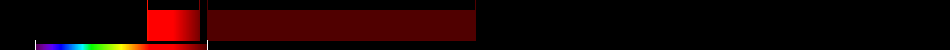Ashburn Vegetation Index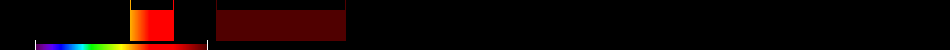Atmospherically Resistant Vegetation Index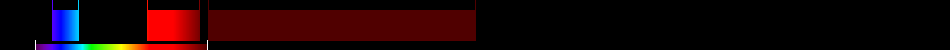Atmospherically Resistant Vegetation Index 2Average reflectance 750 to 850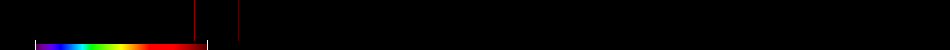Blue-wide dynamic range vegetation index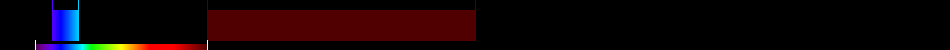Canopy Chlorophyll Content Index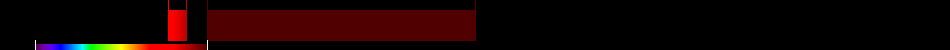Chlorophyll Index Green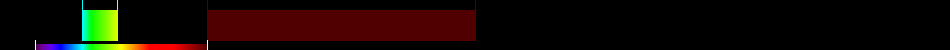Chlorophyll IndexRedEdgeChlorophyll vegetation index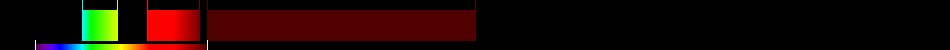Coloration Index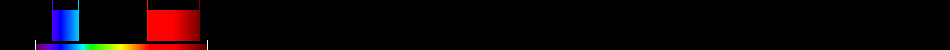Corrected Transformed Vegetation Index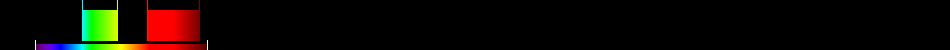CRI550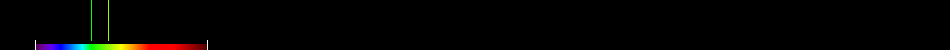Difference NIR/GreenDifferenced Vegetation Index MSSEnhanced Vegetation Index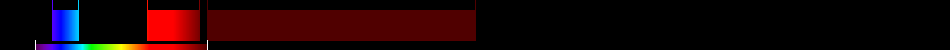Enhanced Vegetation Index 2Enhanced Vegetation Index 2Ferric iron, Fe3+Global Environment Monitoring IndexGreen atmospherically resistant vegetation index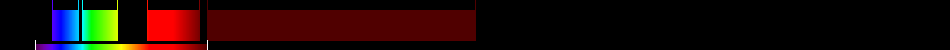Green leaf index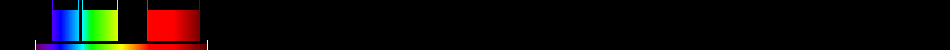Green Normalized Difference Vegetation Index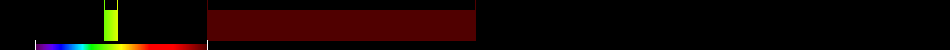Green Optimized Soil Adjusted Vegetation IndexGreen-Blue NDVI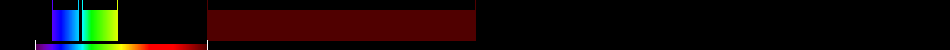Green-Red NDVIHueIdeal vegetation indexInfrared percentage vegetation indexIntensityInverse reflectance 550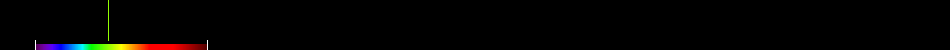Log RatiomCRIG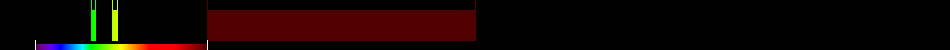Misra Green Vegetation Index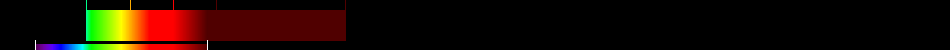Misra Non Such IndexMisra Soil Brightness IndexMisra Yellow Vegetation IndexModified Simple Ratio NIR/REDNonlinear vegetation indexNorm GNorm NIRNorm RNormalized Difference 415/435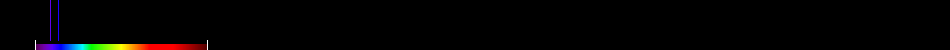Normalized Difference 550/450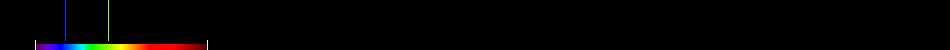Normalized Difference 750/550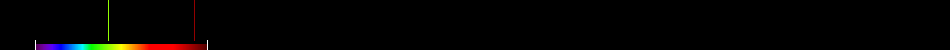Normalized Difference Green/RedNormalized Difference NIR/BlueNormalized Difference NIR/GreenNormalized Difference NIR/RededgeNormalized Difference Red/GreenPan NDVIRed-Blue NDVIShape IndexSimple Ratio 440/740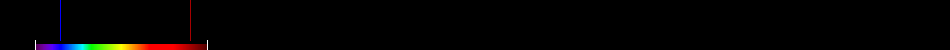Simple Ratio 450/550Simple Ratio 520/420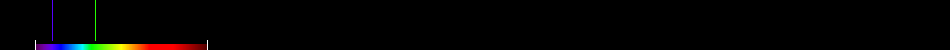Simple Ratio 550/420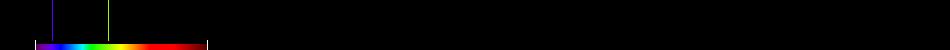Simple Ratio 556/750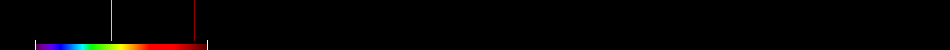Simple Ratio 750/550Simple Ratio 750/555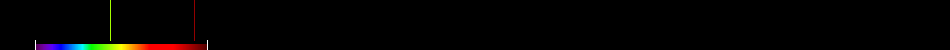Simple Ratio 860/550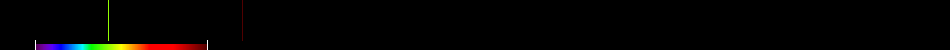Simple Ratio NIR/GSimple Ratio NIR/REDSimple Ratio NIR/RededgeSimple Ratio Red/BlueSimple Ratio Red/GreenSimple Ratio Red/NIRSingle Band 495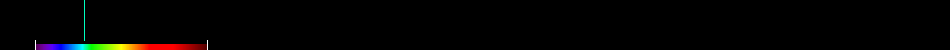Single Band 550Single Band 555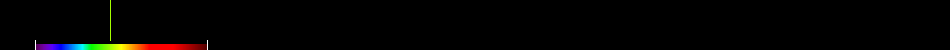Single Band 735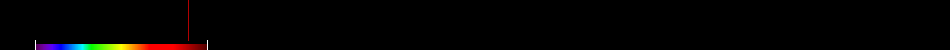Single Band 850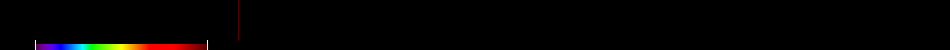Single Band 885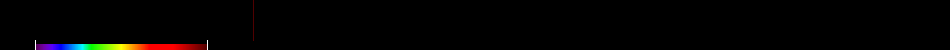Soil and Atmospherically Resistant Vegetation Index 2Soil Background LineSQRT(IR/R)Tasselled Cap - Green Vegetation Index MSSTasselled Cap - Non Such Index MSSTasselled Cap - Soil Brightness Index MSSTasselled Cap - Yellow Vegetation Index MSSTransformed NDVITransformed Soil Adjusted Vegetation Index 2Transformed Vegetation IndexVisible Atmospherically Resistant Index Green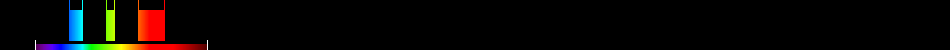Weighted Difference Vegetation IndexWide Dynamic Range Vegetation Index